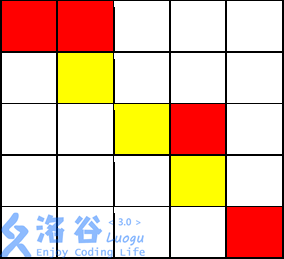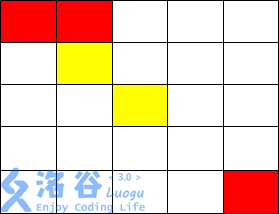# P3956 棋盘

• 8.5K通过
• 38.7K提交
• 题目提供者 CCF_NOI
• 评测方式 云端评测
• 标签 剪枝 广度优先搜索,BFS 搜索 深度优先搜索,DFS NOIp普及组 2017
• 难度 普及/提高-
• 时空限制 1000ms / 256MB
• 提示：收藏到任务计划后，可在首页查看。

## 题目描述

有一个$m \times m$的棋盘，棋盘上每一个格子可能是红色、黄色或没有任何颜色的。你现在要从棋盘的最左上角走到棋盘的最右下角。

任何一个时刻，你所站在的位置必须是有颜色的（不能是无色的）， 你只能向上、 下、左、 右四个方向前进。当你从一个格子走向另一个格子时，如果两个格子的颜色相同，那你不需要花费金币；如果不同，则你需要花费 $1$个金币。

另外， 你可以花费 $2$ 个金币施展魔法让下一个无色格子暂时变为你指定的颜色。但这个魔法不能连续使用， 而且这个魔法的持续时间很短，也就是说，如果你使用了这个魔法，走到了这个暂时有颜色的格子上，你就不能继续使用魔法； 只有当你离开这个位置，走到一个本来就有颜色的格子上的时候，你才能继续使用这个魔法，而当你离开了这个位置（施展魔法使得变为有颜色的格子）时，这个格子恢复为无色。

现在你要从棋盘的最左上角，走到棋盘的最右下角，求花费的最少金币是多少？

## 输入输出格式

输入格式：

第一行包含两个正整数$m, n$，以一个空格分开，分别代表棋盘的大小，棋盘上有颜色的格子的数量。

接下来的$n$行，每行三个正整数$x, y, c$， 分别表示坐标为$(x,y)$的格子有颜色$c$。

其中$c=1$ 代表黄色，$c=0$ 代表红色。 相邻两个数之间用一个空格隔开。 棋盘左上角的坐标为$(1, 1)$，右下角的坐标为$( m, m)$。

棋盘上其余的格子都是无色。保证棋盘的左上角，也就是$(1, 1)$ 一定是有颜色的。

输出格式：

一个整数，表示花费的金币的最小值，如果无法到达，输出$-1$。

## 输入输出样例

输入样例#1： 复制
5 7
1 1 0
1 2 0
2 2 1
3 3 1
3 4 0
4 4 1
5 5 0
输出样例#1： 复制
8
输入样例#2： 复制
5 5
1 1 0
1 2 0
2 2 1
3 3 1
5 5 0
输出样例#2： 复制
-1

## 说明

### 输入输出样例 1 说明从$(1,1)$开始，走到$(1,2)$不花费金币

从$(1,2)$向下走到$(2,2)$花费 $1$ 枚金币

从$(2,2)$施展魔法，将$(2,3)$变为黄色，花费 $2$ 枚金币

从$(2,2)$走到$(2,3)$不花费金币

从$(2,3)$走到$(3,3)$不花费金币

从$(3,3)$走到$(3,4)$花费 $1$ 枚金币

从$(3,4)$走到$(4,4)$花费 $1$ 枚金币

从$(4,4)$施展魔法，将$(4,5)$变为黄色，花费$2$ 枚金币，

从$(4,4)$走到$(4,5)$不花费金币

从$(4,5)$走到$(5,5)$花费 $1$ 枚金币

共花费 $8$枚金币。

### 输入输出样例 2 说明从$( 1, 1)$走到$( 1, 2)$,不花费金币

从$( 1, 2)$走到$( 2, 2)$,花费$1$金币

施展魔法将$( 2, 3)$变为黄色,并从$( 2, 2)$走到$( 2, 3)$花费$2$ 金币

从$( 2, 3)$走到$( 3, 3)$不花费金币

从$( 3, 3)$只能施展魔法到达$( 3, 2),( 2, 3),( 3, 4),( 4, 3)$

而从以上四点均无法到达$( 5, 5)$,故无法到达终点,输出$-1$

### 数据规模与约定

对于 $30\%$的数据, $1 ≤ m ≤ 5, 1 ≤ n ≤ 10$。

对于 $60\%$的数据, $1 ≤ m ≤ 20, 1 ≤ n ≤ 200$。

对于 $100\%$的数据, $1 ≤ m ≤ 100, 1 ≤ n ≤ 1,000$。

提示
标程仅供做题后或实在无思路时参考。
请自觉、自律地使用该功能并请对自己的学习负责。
如果发现恶意抄袭标程，将按照I类违反进行处理。